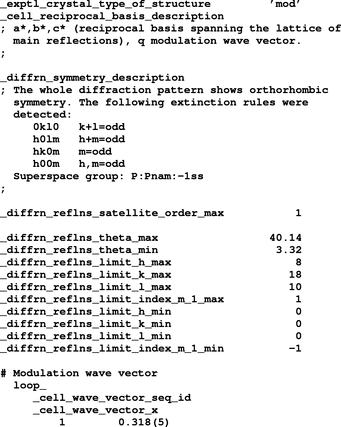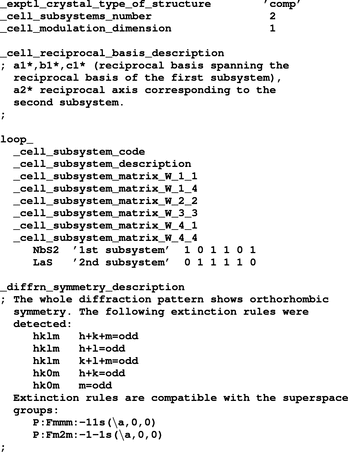International
Tables for
Crystallography
Volume G
Definition and exchange of crystallographic data
Edited by S. R. Hall and B. McMahon

International Tables for Crystallography (2006). Vol. G. ch. 3.4, p. 137

## Section 3.4.4.1. Description of reciprocal space

#### 3.4.4.1. Description of reciprocal space

| top | pdf |

Modulated and composite structures need more than three reciprocal vectors in order to index the whole set of reflections with integer numbers. Hence a diffraction vector is written aswhere the notation has been chosen according to the core CIF dictionary. In the case of a modulated structure,,andare the reciprocal vectors of the reference structure (and therefore h, k and l index the main reflections).are the modulation wave vectors. They are three-dimensional vectors with some irrational component (if the modulated structure is incommensurate) in the lattice spanned by,and. d is the dimension of the modulation. In the case of composite structures, the diffraction pattern can be indexed using 3 + d (arbitrarily selected) vectors.,andnormally span the reciprocal lattice of the main reflections of one of the substructures (notice that this is only one particular, but highly intuitive, choice). The remaining d vectors withare the wave vectors of the modulation [in equation (3.4.4.1)].

In a composite structure, the (3 + d)-dimensional reciprocal basis of the subsystemis determined by a (3 + d) × (3 + d) matrix[see van Smaalen (1995) and references therein]:where the subscripts i = 1, 2 and 3 label the reciprocal vectors,and, andlabel the wave vectors of the modulation expressed as linear combinations of,and.

The simplest case corresponds to a one-dimensional (d = 1) modulated structure. Consider for example the incommensurate phase of K2SeO4. The wave vector of the modulation can be chosen to be. Relevant information about the diffraction pattern of this compound is expressed using both the core CIF and msCIF dictionaries as shown in Example 3.4.4.1.

#### Example 3.4.4.1. msCIF description of the diffraction pattern of a one-dimensional modulated structure.A more complicated example is the composite structure (LaS)1.14NbS2. The two mutually incommensurate subsystems (along the a axis) are (van Smaalen, 1991) NbS2 () and LaS (). The reciprocal basis can be chosen to be,,and. For this particular choice, the two W matrices [see equation (3.4.4.2)] areThis information is transcribed to CIF format as shown in Example 3.4.4.2. (Note that the default values for the wave vector components and the elements of W are 0.)

#### Example 3.4.4.2. Representation of two mutually incommensurate subsystems.### ReferencesSmaalen, S. van (1991). Superspace-group approach to the modulated structure of the inorganic misfit layer compound (LaS)1.14NbS2. J. Phys. Condens. Matter, 3, 1247–1263.Google ScholarSmaalen, S. van (1995). Incommensurate crystal structures. Crystallogr. Rev. 4, 79–202.Google Scholar